2 Analyzing TwoVariable Data Lesson 2 7 Assessing

• Slides: 152 Analyzing Two-Variable Data Lesson 2. 7 Assessing a Regression Model Statistics and Probability with Applications, 3 rd Edition Starnes & Tabor Bedford Freeman Worth PublishersAssessing a Regression Model Learning Targets After this lesson, you should be able to: ü Use a residual plot to determine if a regression model is appropriate. ü Interpret the standard deviation of the residuals. ü Interpret r 2. Statistics and Probability with Applications, 3 rd Edition 2Assessing a Regression Model Now that we have learned how to calculate a least-squares regression line, it is important to assess how well the line fits the data. We do this by asking two questions: • Is a line the right model to use, or would a curve be better? • If a line is the right model to use, how well does it make predictions? We can use residuals to assess whether a regression model is appropriate by making a residual plot. Residual Plot A residual plot is a scatterplot that plots the residuals on the vertical axis and the explanatory variable on the horizontal axis. Statistics and Probability with Applications, 3 rd Edition 3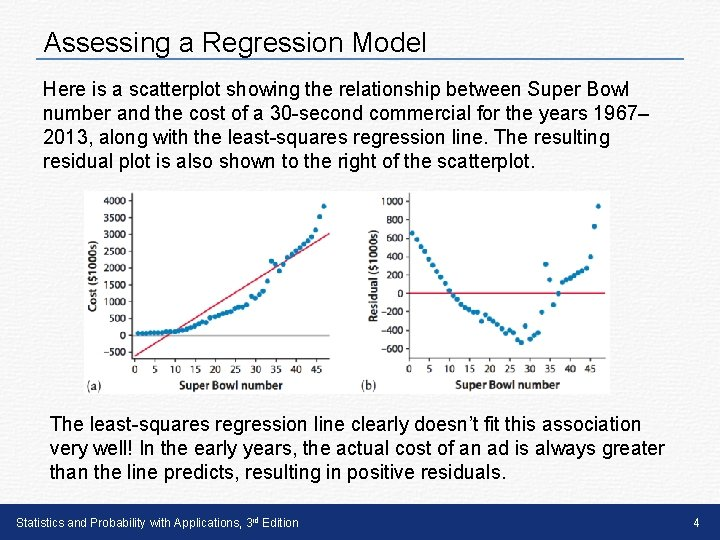Assessing a Regression Model Here is a scatterplot showing the relationship between Super Bowl number and the cost of a 30 -second commercial for the years 1967– 2013, along with the least-squares regression line. The resulting residual plot is also shown to the right of the scatterplot. The least-squares regression line clearly doesn’t fit this association very well! In the early years, the actual cost of an ad is always greater than the line predicts, resulting in positive residuals. Statistics and Probability with Applications, 3 rd Edition 4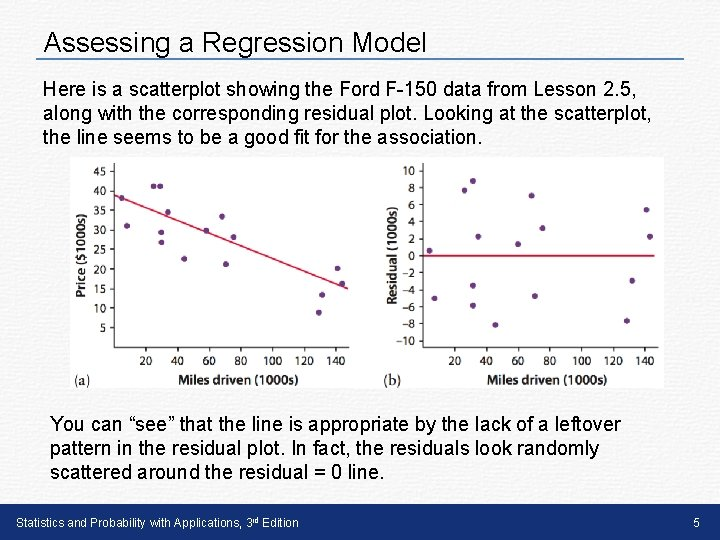Assessing a Regression Model Here is a scatterplot showing the Ford F-150 data from Lesson 2. 5, along with the corresponding residual plot. Looking at the scatterplot, the line seems to be a good fit for the association. You can “see” that the line is appropriate by the lack of a leftover pattern in the residual plot. In fact, the residuals look randomly scattered around the residual = 0 line. Statistics and Probability with Applications, 3 rd Edition 5Assessing a Regression Model Interpreting a Residual Plot To determine if the regression model is appropriate, look at the residual plot. • If there is no leftover pattern in the residual plot, the regression model is appropriate. • If there is a leftover pattern in the residual plot, the regression model is not appropriate. Statistics and Probability with Applications, 3 rd Edition 6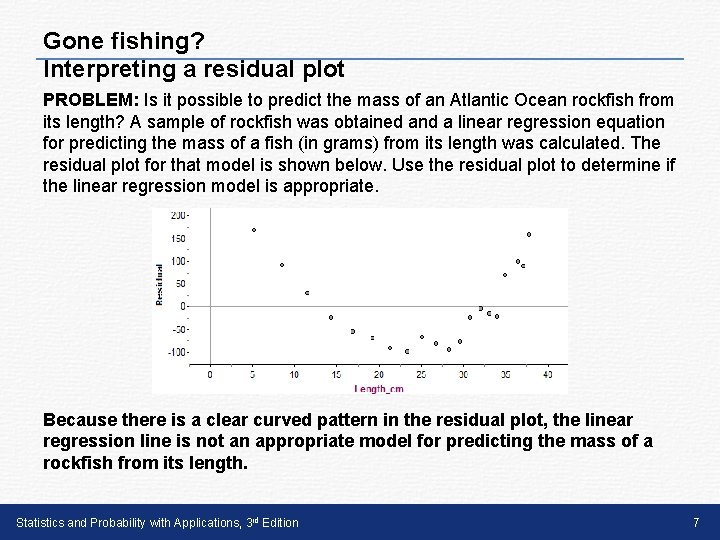Gone fishing? Interpreting a residual plot PROBLEM: Is it possible to predict the mass of an Atlantic Ocean rockfish from its length? A sample of rockfish was obtained and a linear regression equation for predicting the mass of a fish (in grams) from its length was calculated. The residual plot for that model is shown below. Use the residual plot to determine if the linear regression model is appropriate. Because there is a clear curved pattern in the residual plot, the linear regression line is not an appropriate model for predicting the mass of a rockfish from its length. Statistics and Probability with Applications, 3 rd Edition 7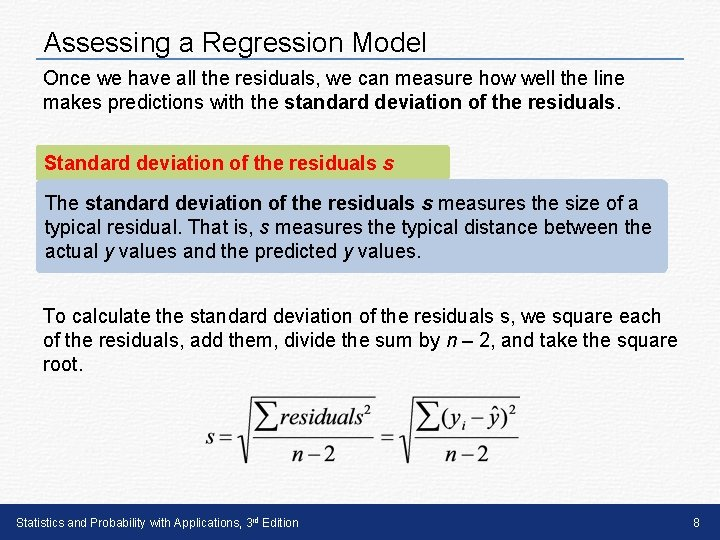Assessing a Regression Model Once we have all the residuals, we can measure how well the line makes predictions with the standard deviation of the residuals. Standard deviation of the residuals s The standard deviation of the residuals s measures the size of a typical residual. That is, s measures the typical distance between the actual y values and the predicted y values. To calculate the standard deviation of the residuals s, we square each of the residuals, add them, divide the sum by n – 2, and take the square root. Statistics and Probability with Applications, 3 rd Edition 8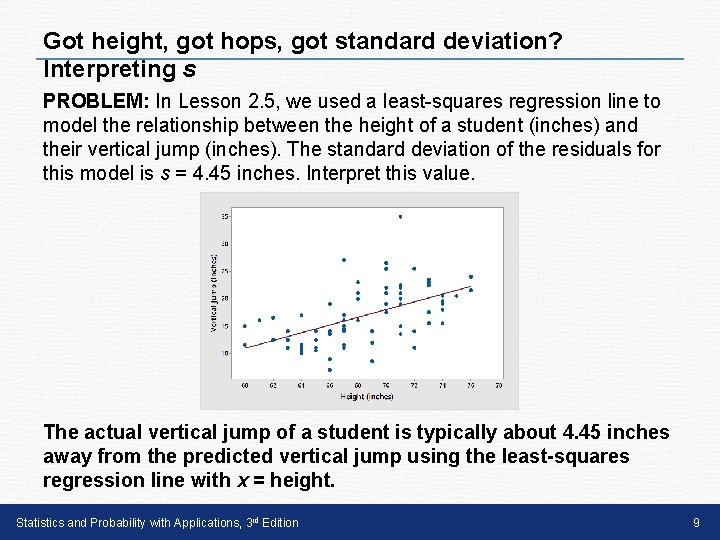Got height, got hops, got standard deviation? Interpreting s PROBLEM: In Lesson 2. 5, we used a least-squares regression line to model the relationship between the height of a student (inches) and their vertical jump (inches). The standard deviation of the residuals for this model is s = 4. 45 inches. Interpret this value. The actual vertical jump of a student is typically about 4. 45 inches away from the predicted vertical jump using the least-squares regression line with x = height. Statistics and Probability with Applications, 3 rd Edition 9Assessing a Regression Model Besides the standard deviation of the residuals s, we can also use the coefficient of determination r 2 ￼ to measure how well the regression line makes predictions. Coefficient of Determination r 2 The coefficient of determination r 2￼ measures the percent reduction in the sum of squared residuals when using the leastsquares regression line to make predictions rather than the mean value of y. In other words, r 2 measures the percent of the variability in the response variable that is accounted for by the least-squares regression line. Statistics and Probability with Applications, 3 rd Edition 10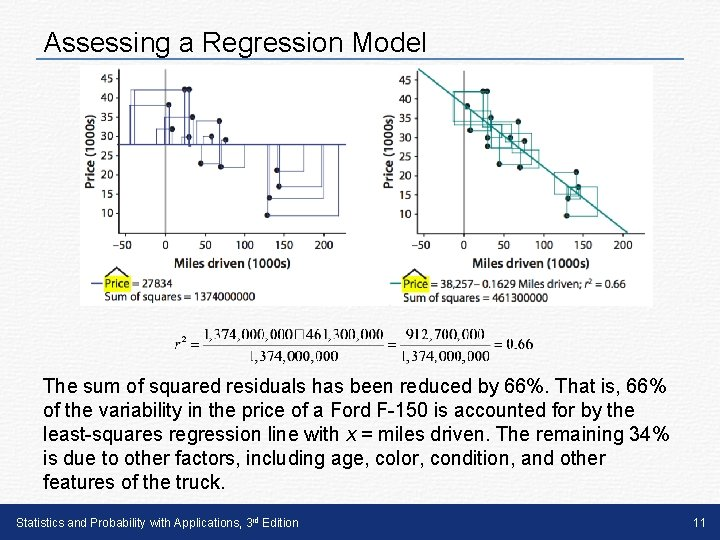Assessing a Regression Model The sum of squared residuals has been reduced by 66%. That is, 66% of the variability in the price of a Ford F-150 is accounted for by the least-squares regression line with x = miles driven. The remaining 34% is due to other factors, including age, color, condition, and other features of the truck. Statistics and Probability with Applications, 3 rd Edition 11PROBLEM: In Lesson 2. 6, we used a least-squares regression line to model the relationship between price and miles driven for 41 used Dodge Chargers. Interpret the value r 2 = 67% for this model. 67% of the variability in the price of a Dodge Charger is accounted for by the least-squares regression line with x = miles driven. Statistics and Probability with Applications, 3 rd Edition 12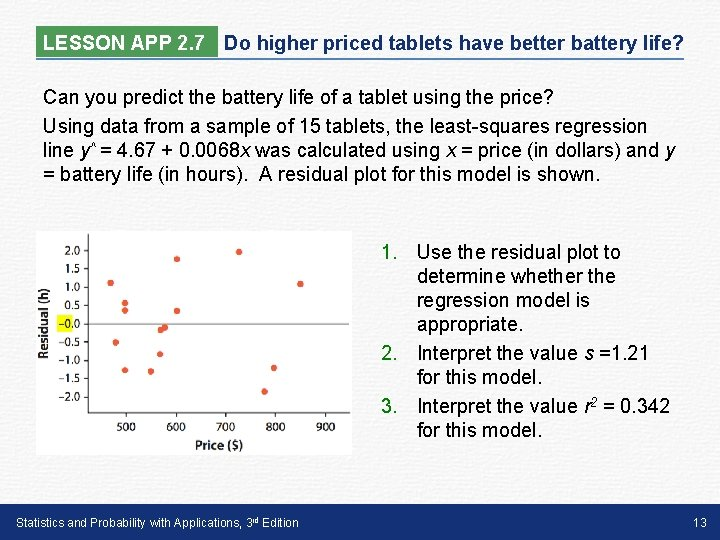LESSON APP 2. 7 Do higher priced tablets have better battery life? Can you predict the battery life of a tablet using the price? Using data from a sample of 15 tablets, the least-squares regression line y^ = 4. 67 + 0. 0068 x was calculated using x = price (in dollars) and y = battery life (in hours). A residual plot for this model is shown. 1. Use the residual plot to determine whether the regression model is appropriate. 2. Interpret the value s =1. 21 for this model. 3. Interpret the value r 2 = 0. 342 for this model. Statistics and Probability with Applications, 3 rd Edition 13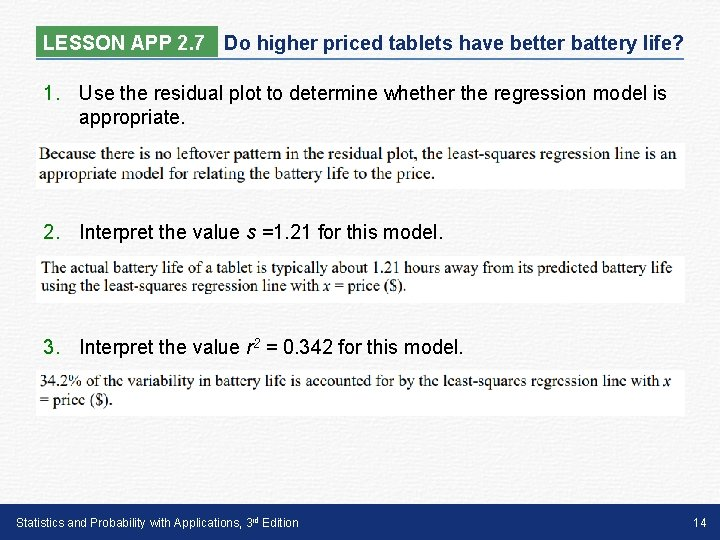LESSON APP 2. 7 Do higher priced tablets have better battery life? 1. Use the residual plot to determine whether the regression model is appropriate. 2. Interpret the value s =1. 21 for this model. 3. Interpret the value r 2 = 0. 342 for this model. Statistics and Probability with Applications, 3 rd Edition 14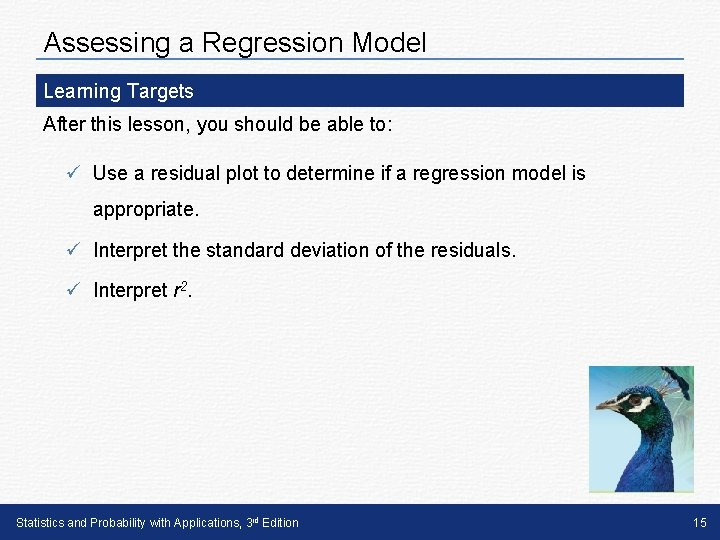Assessing a Regression Model Learning Targets After this lesson, you should be able to: ü Use a residual plot to determine if a regression model is appropriate. ü Interpret the standard deviation of the residuals. ü Interpret r 2. Statistics and Probability with Applications, 3 rd Edition 15